﻿ 模糊直方图模型的运动目标跟踪
«上一篇文章快速检索 高级检索

 智能系统学报2019, Vol. 14Issue (5): 939-946  DOI: 10.11992/tis.2018070330

### 引用本文XIU Chunbo, LI Xin, BA Fushan. Target tracking based on the fuzzy histogram model[J]. CAAI Transactions on Intelligent Systems, 2019, 14(5): 939-946. DOI: 10.11992/tis.201807033.### 文章历史

1. 天津工业大学 电气工程与自动化学院，天津 300387;
2. 天津工业大学 电工电能新技术天津市重点实验室，天津 300387

Target tracking based on the fuzzy histogram model
XIU Chunbo 1,2, LI Xin 1, BA Fushan 1
1. School of Electrical Engineering and Automation, Tianjin Polytechnic University, Tianjin 300387, China;
2. Key Laboratory of Advanced Electrical Engineering and Energy Technology, Tianjin Polytechnic University, Tianjin 300387, China
Abstract: To enhance the robustness of the tracking system to the hue drift and illumination variation, a new target model based on the fuzzy histogram is proposed in this paper. First, some fuzzy ranks were defined in the hue domain, and the fuzzy histogram model of the target area is built according to the fuzzy membership degree to reduce the sensibility to the hue thresholds. Then, the probability distribution image of the tracking-scene image based on the back-projection of the fuzzy histogram model was built. Finally, the Camshift tracking method was used to perform target recognition, location, and tracking. The simulation results show that the method has better adaptability to the interference caused by hue drift than conventional methods. Target tracking can still be completed by the method when the target moves in the illumination-varying condition, such as front, side, and back light. The average tracking computation time of a single frame of the method is similar to that of the basic Camshift method. The maximum tracking computation time of a single frame is less than 40 ms, which can satisfy the real-time request of tracking systems such as a television tracking system.
Key words: fuzzy histogram    target tracking    illumination variation    hue drift    back-projection    real-time tracking    membership degree    target location

Meanshift算法、Camshift算法以及各种改进方法均是利用目标的色度(Hue)直方图模型来描述目标的基本特征，利用反向投影实现目标的定位跟踪[12-18]。因此目标直方图模型的稳定性和准确性直接影响着跟踪性能。现有Camshift等跟踪方法采用精确集合的定义选取划分阈值[12-18]，而实际跟踪过程中，由于光照变化等干扰因素会造成场景图像的色度产生一定的漂移。从而引起目标直方图模型的不稳定，降低了目标跟踪的性能。

1 模糊直方图模型

 ${q'_u} = \sum\limits_{i = 1}^s {\delta [b({x_i},{y_i}) - u]}$ (1)

 {\mu _k}(h) = \left\{ \begin{aligned} & \frac{{h - {h_{k - 2}}}}{{{h_{k - 1}} - {h_{k - 2}}}},\quad{h_{k - 2}} < h \leqslant {h_{k - 1}} \\ & 1,\quad{h_{k - 1}} < h \leqslant {h_k} \\ & \frac{{{h_{k + 1}} - h}}{{{h_{k + 1}} - {h_k}}},\quad{h_k} < h \leqslant {h_{k + 1}} \\ & 0,\quad h \leqslant {h_{k - 2}} \text{或} h > {h_{k + 1}} \end{aligned} \right.

 ${q_u} = \sum\limits_{i = 1}^s {{\mu _u}h({x_i},{y_i})}$ (2)

2 基于模糊直方图模型的跟踪方法

 $p(x,y) ={\rm{INT}} \left[ {\frac{{\displaystyle\sum\limits_{u = 1}^m {{\mu _u}h(x,y) \cdot {q_u} \times 255} }}{{\max \{ {q_j}|j = 1,2,\cdots,m\} \displaystyle\sum\limits_{u = 1}^m {{\mu _u}[h(x,y)]} }}} \right]$ (3)

1)选定跟踪目标区域，利用式(2)建立目标区域的色度模糊直方图模型；

2)获取当前跟踪场景，利用式(3)求取当前跟踪场景的反向投影概率分布图；

3)根据场景反向投影概率分布图中各像素点的灰度值，求取跟踪搜索窗的质心坐标(xcyc)，即

 ${x_c} = \frac{{\displaystyle\sum\limits_{x,y} {x \cdot p(x,y)} }}{{\displaystyle\sum\limits_{x,y} {p(x,y)} }}$
 ${y_c} = \frac{{\displaystyle\sum\limits_{x,y} {y \cdot p(x,y)} }}{{\displaystyle\sum\limits_{x,y} {p(x,y)} }}$

4)自适应调整跟踪搜索窗的边长，即

 $l = 2\sqrt {\sum\limits_{x,y} {p(x,y)/256} }$

5)平移跟踪搜索窗的中心至质心位置，并重复3)~5)，直到移动距离小于设定阈值；

6)更新跟踪目标的长L、宽W及方向角θ，即

 $L = \sqrt {\frac{{A + \sqrt {{B^2} + {C^2}} }}{2}}$
 $W = \sqrt {\frac{{A - \sqrt {{B^2} + {C^2}} }}{2}}$
 $\theta = \frac{1}{2}\arctan \frac{B}{C}$

 $A = \frac{{\displaystyle\sum\limits_{x,y} {p(x,y)({x^2} + {y^2})} }}{{\displaystyle\sum\limits_{x,y} {p(x,y)} }} - (x_c^2 + y_c^2)$
 $B = 2\left[ {\frac{{\displaystyle\sum\limits_{x,y} {xyp(x,y)} }}{{\displaystyle\sum\limits_{x,y} {p(x,y)} }} - {x_c}{y_c}} \right]$
 $C = \frac{{\displaystyle\sum\limits_{x,y} {p(x,y)({x^2} - {y^2})} }}{{\displaystyle\sum\limits_{x,y} {p(x,y)} }} - (x_c^2 - y_c^2)$

7)返回1)，根据新确定的目标区域重建目标模糊直方图模型，对下一帧场景图像进行定位跟踪。

3 实验结果分析 3.1 色度漂移直方图模型比较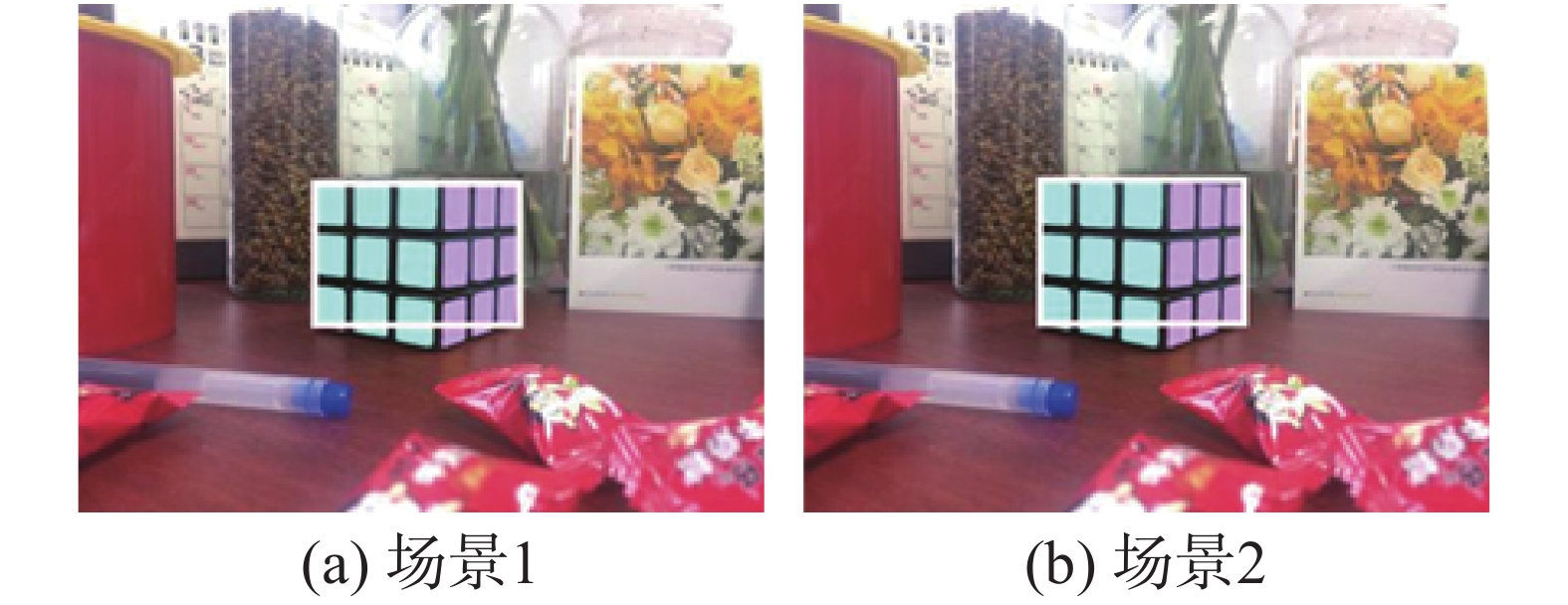Download: 图 1 不同采样时刻场景 Fig. 1 Scenes sampled at different times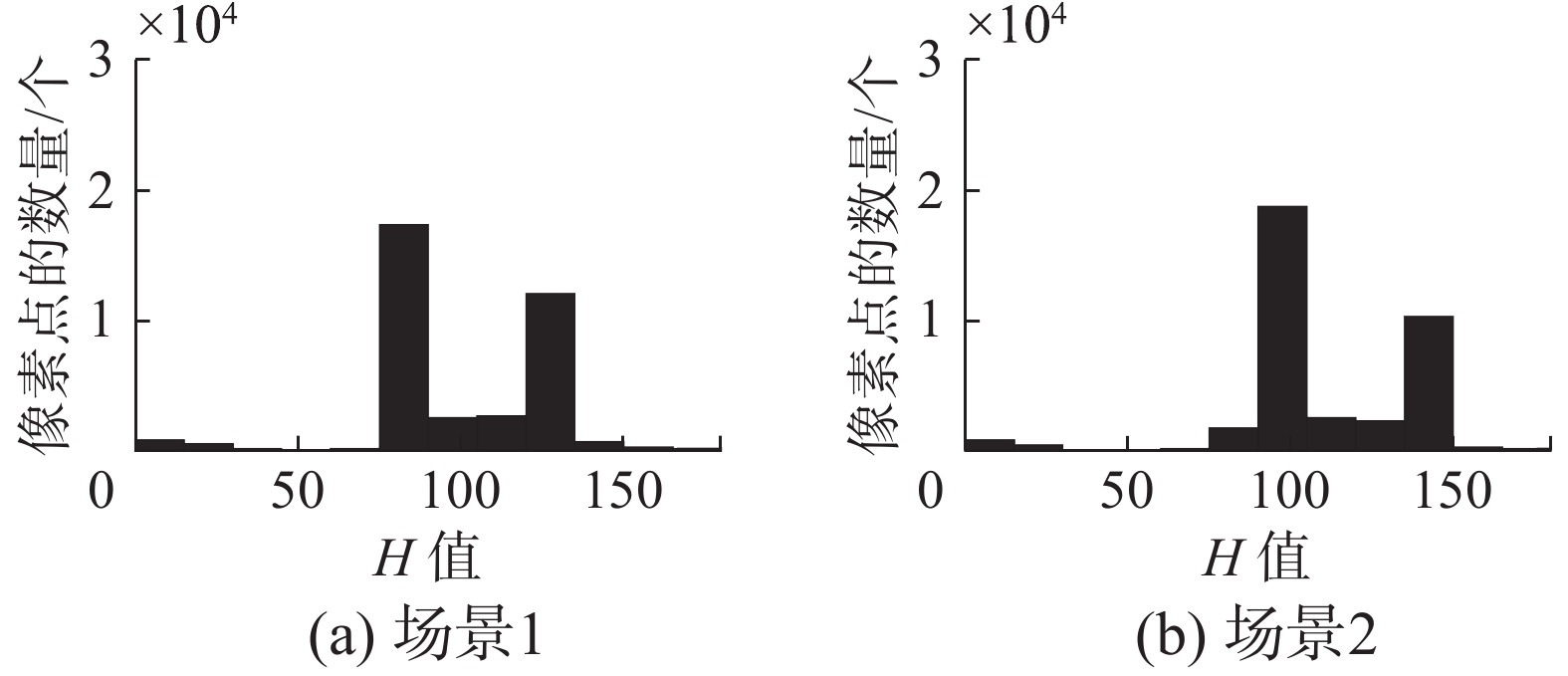Download: 图 2 不同采样时刻场景图中目标直方图 Fig. 2 Target histograms in the scenes sampled at different times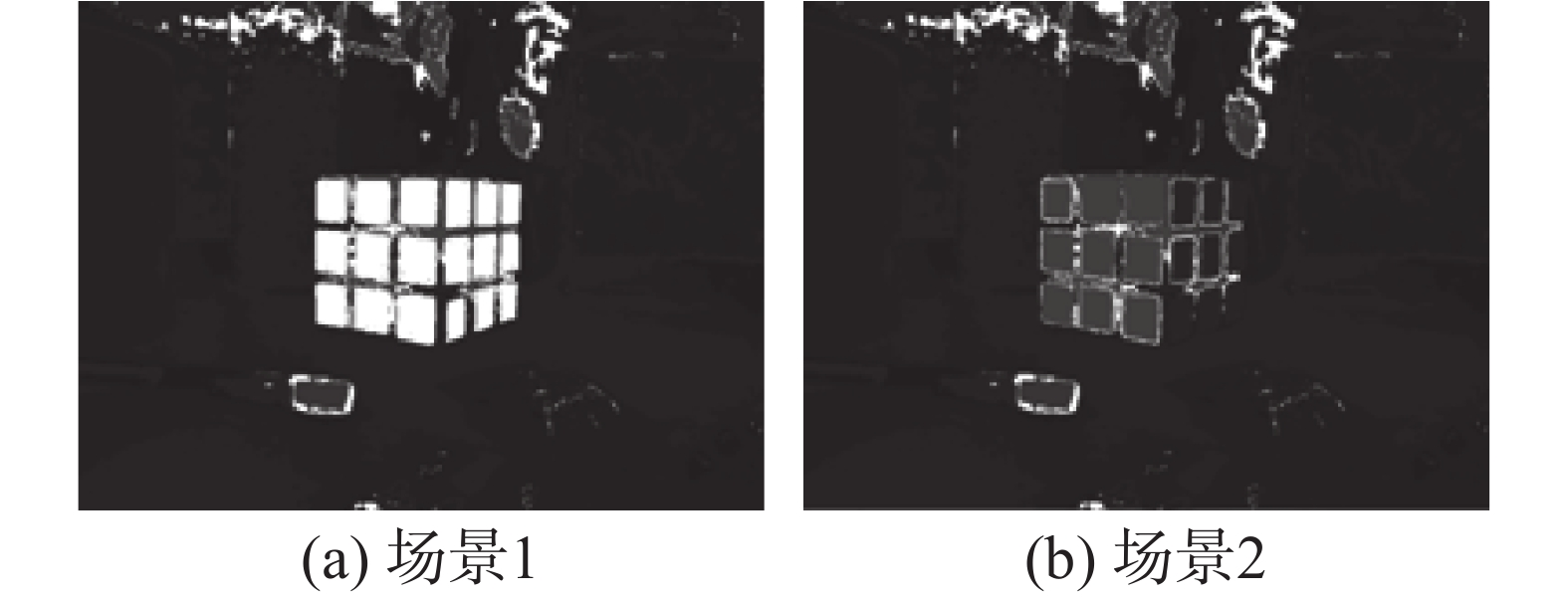Download: 图 3 基于场景1的直方图所得反向投影 Fig. 3 Projection images based on the histogram in scene 1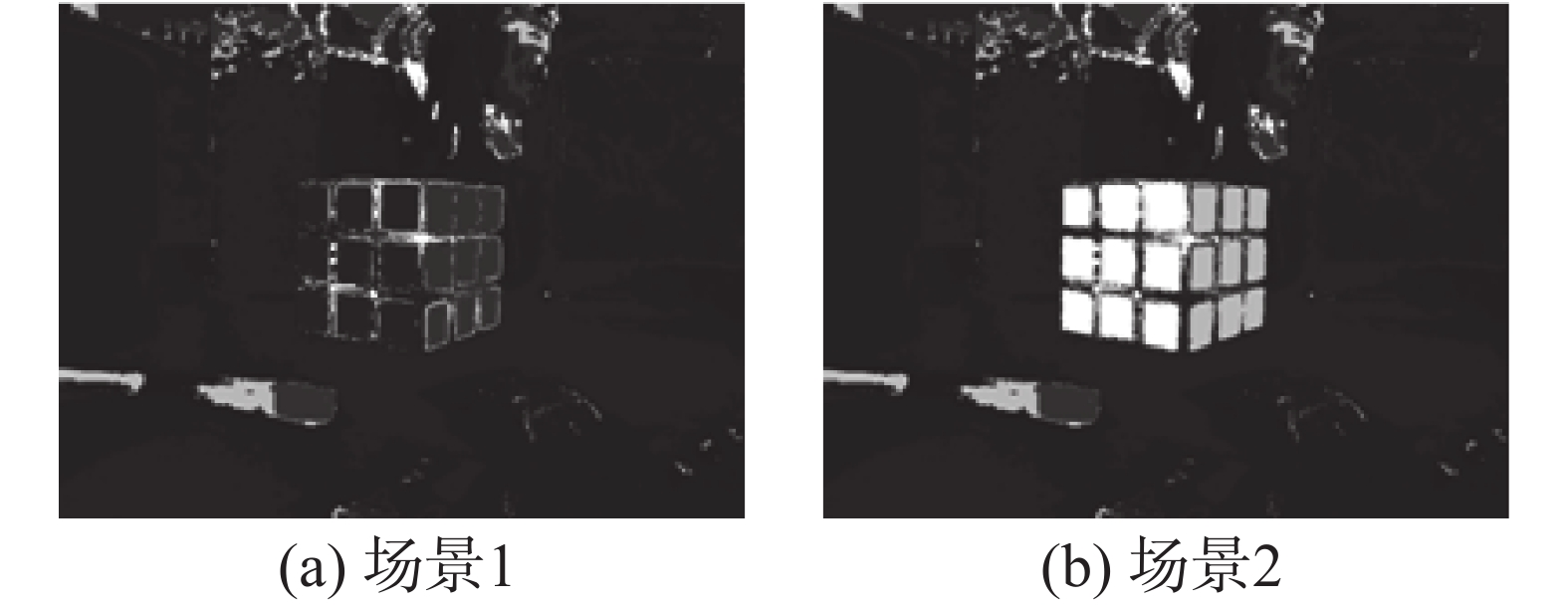Download: 图 4 基于场景2的直方图所得反向投影 Fig. 4 Projection images based on the histogram in scene 2Download: 图 5 不同采样时刻场景图中目标模糊直方图 Fig. 5 Target fuzzy histograms in the scenes samples at different times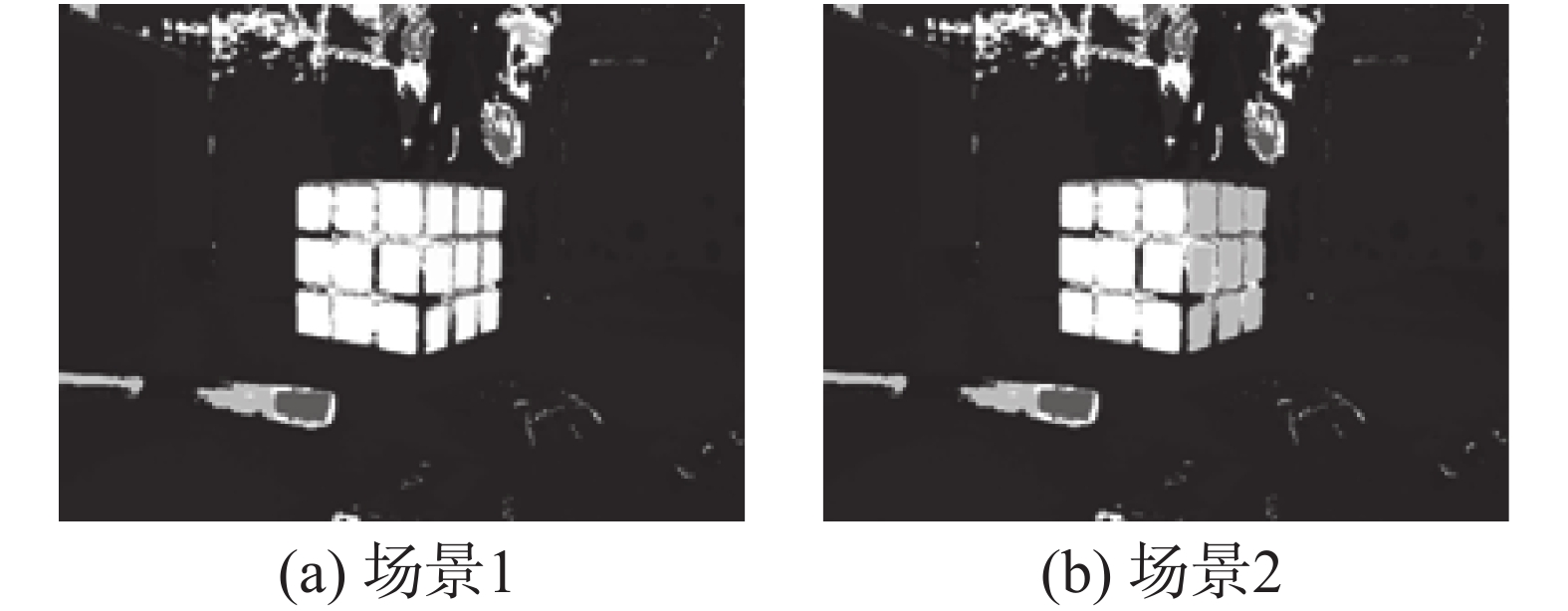Download: 图 6 基于场景1的目标模糊直方图所得反向投影 Fig. 6 Projection images based on the target fuzzy histogram in scene 1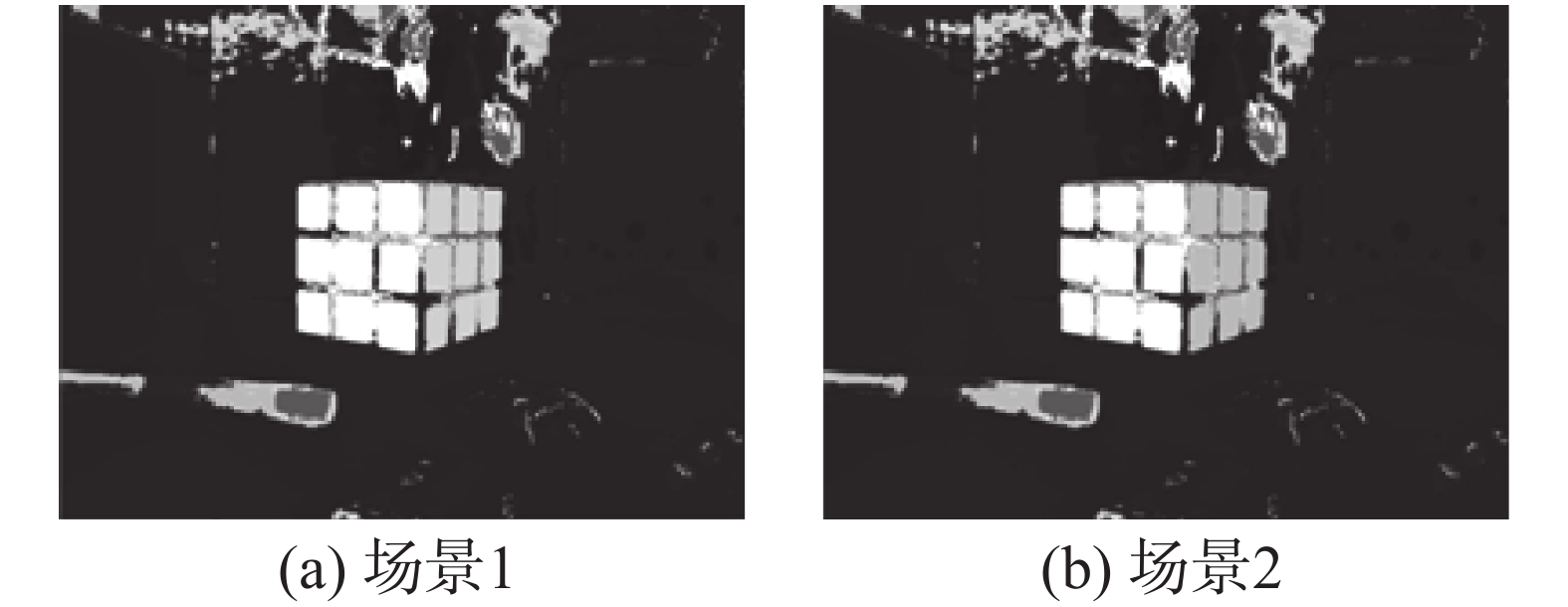Download: 图 7 基于场景2的目标模糊直方图所得反向投影 Fig. 7 Projection images based on the target fuzzy histogram in scene 2
3.2 跟踪实验结果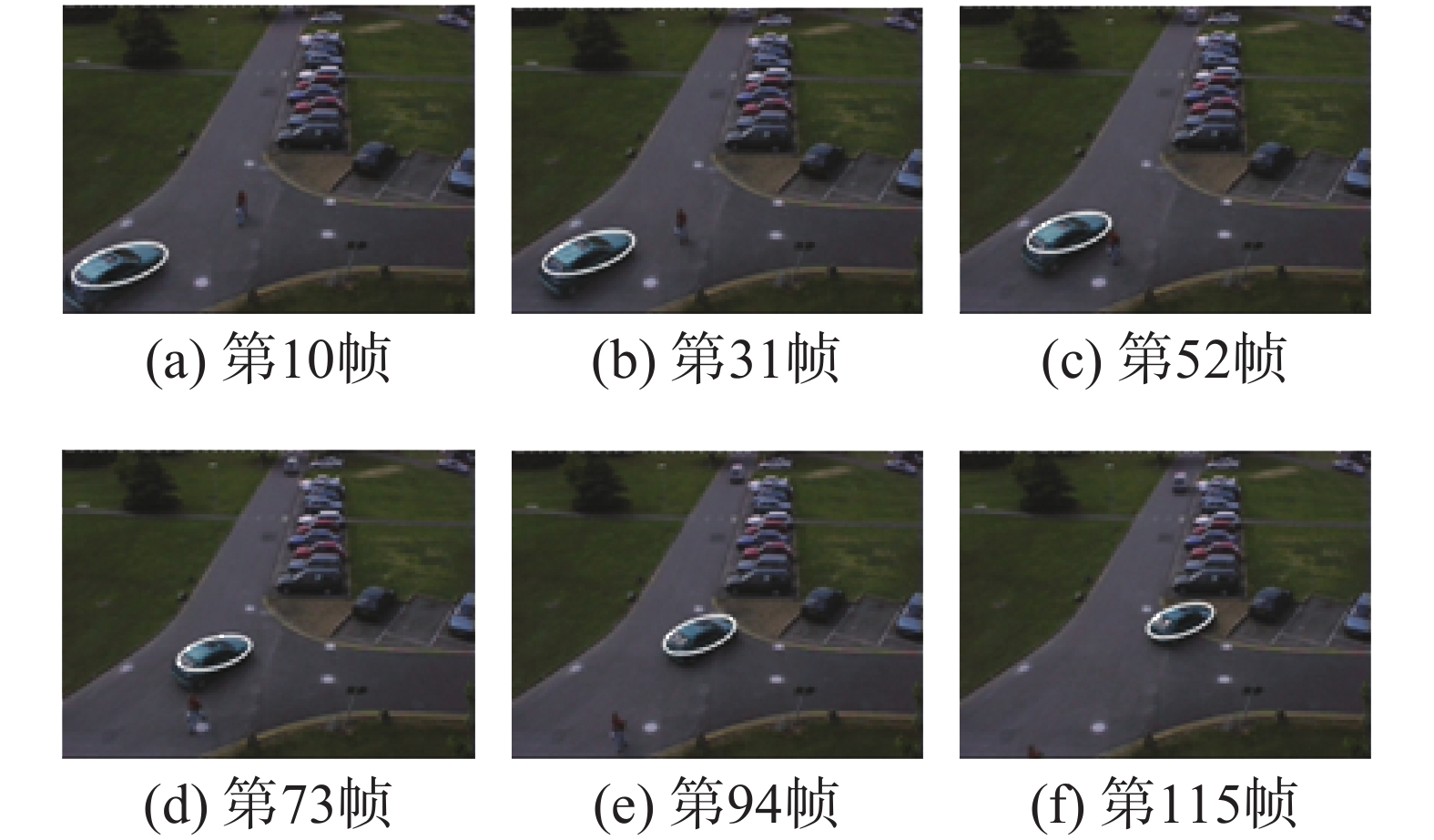Download: 图 8 传统直方图模型跟踪效果 Fig. 8 Tracking result based on the traditionsl histogram model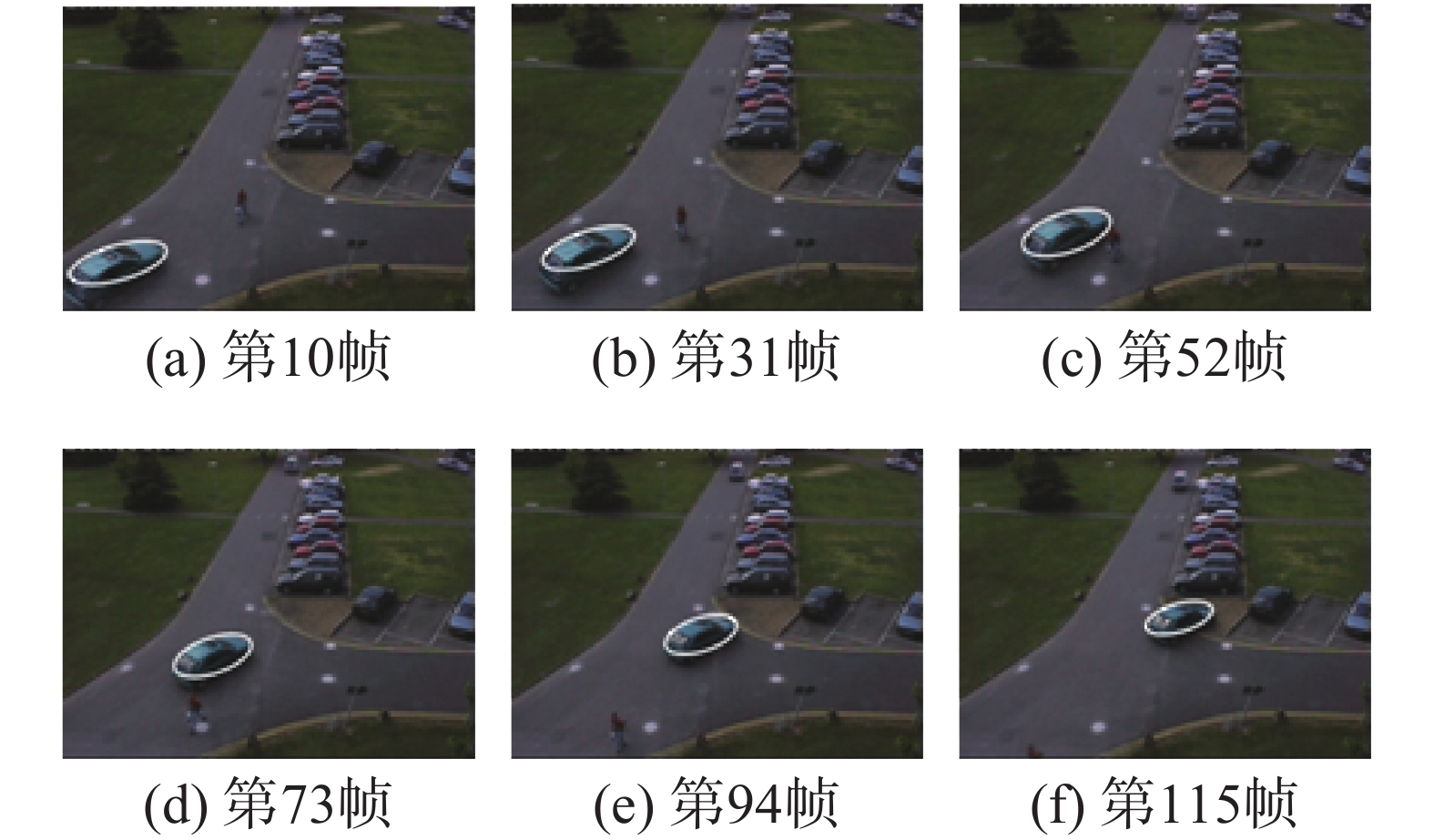Download: 图 9 显著性直方图模型跟踪效果 Fig. 9 Tracking result based on the saliency histogram model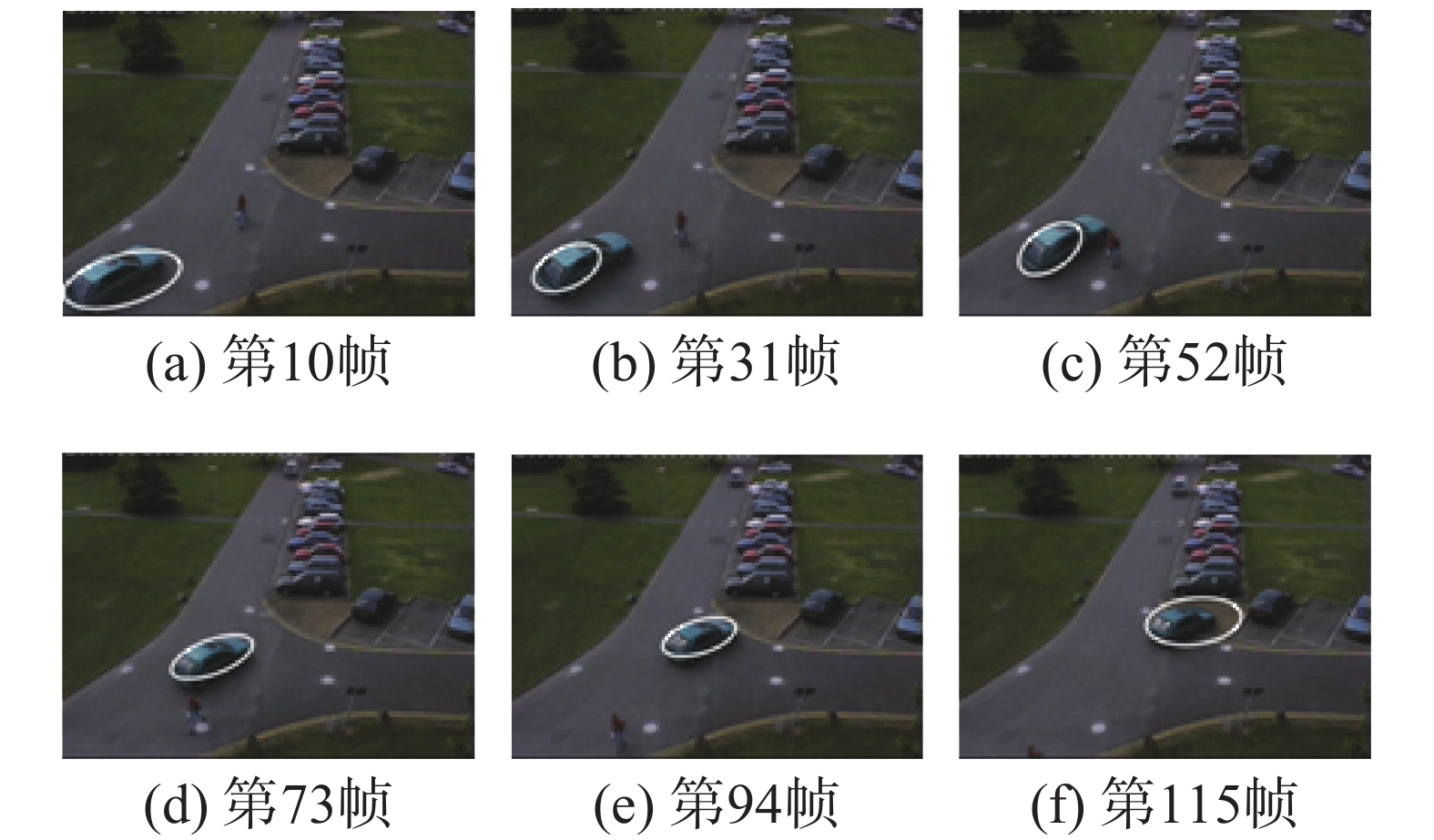Download: 图 10 多特征融合直方图模型跟踪效果 Fig. 10 Tracking result based on multiple features fusion histogram model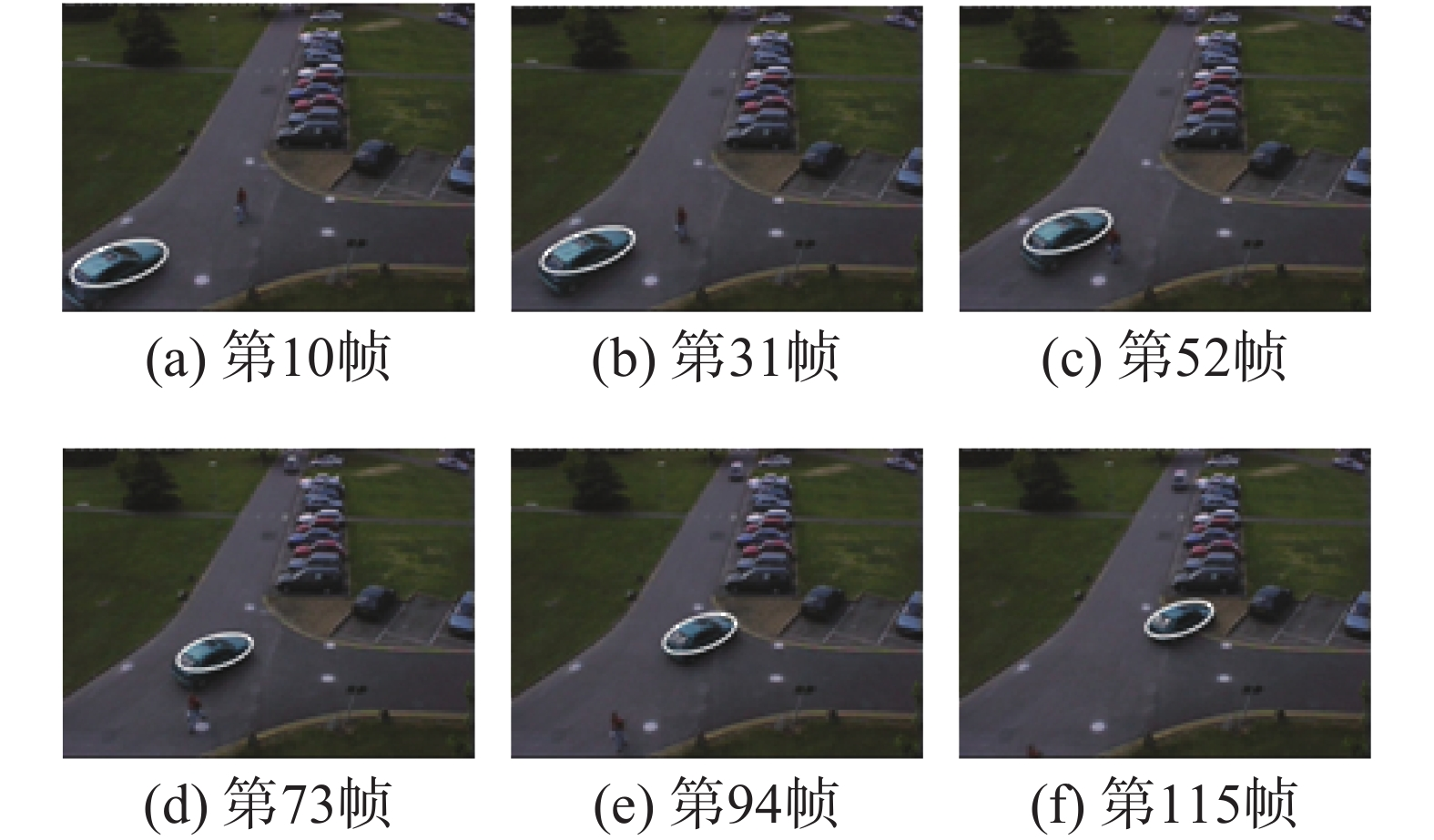Download: 图 11 模糊直方图模型跟踪效果 Fig. 11 Tracking result based on fuzzy histogram model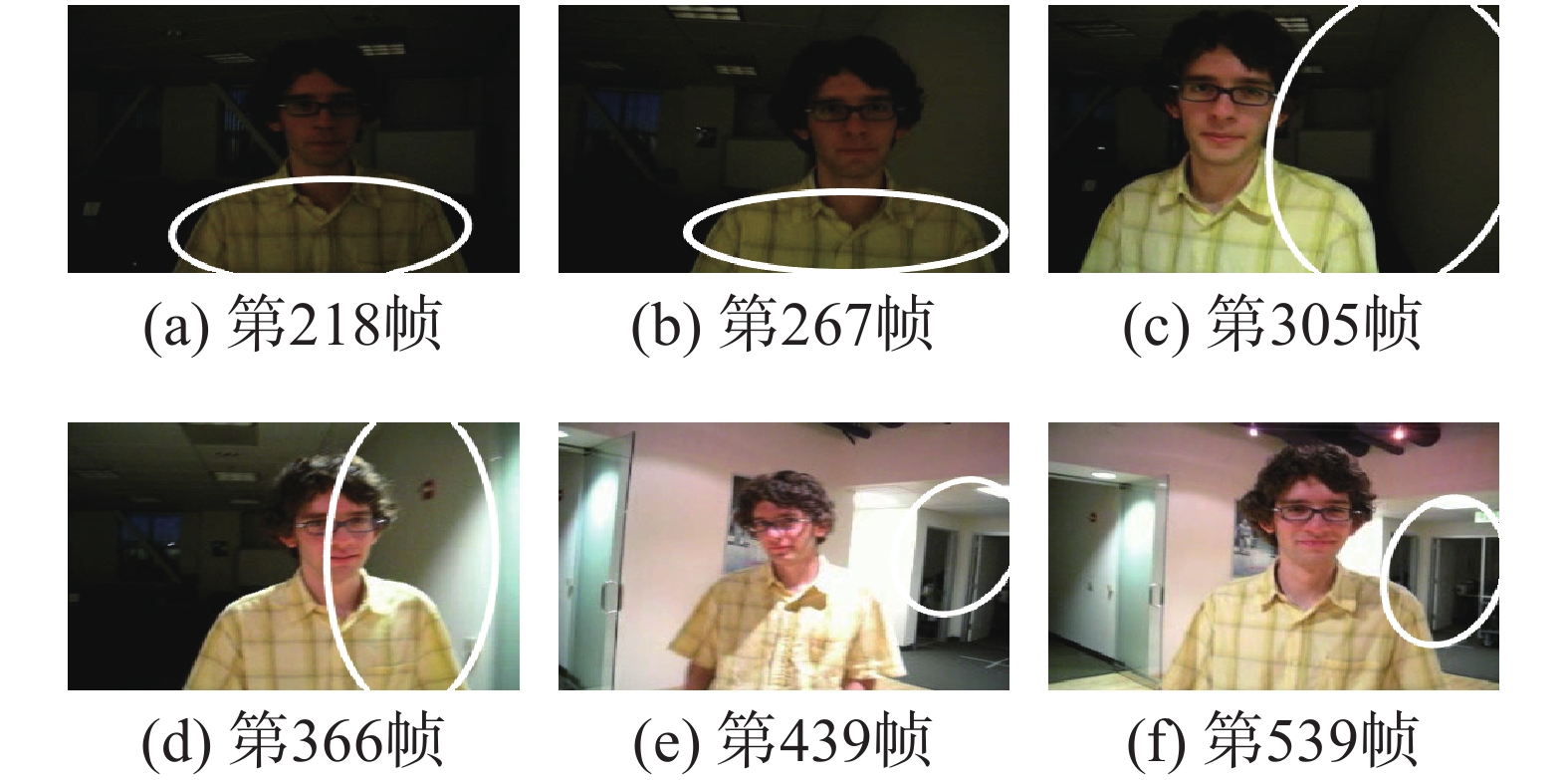Download: 图 12 传统直方图模型跟踪效果 Fig. 12 Tracking result based on the traditional histogram model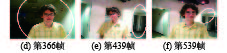Download: 图 13 显著性直方图模型跟踪效果 Fig. 13 Tracking result based on the saliency histogram model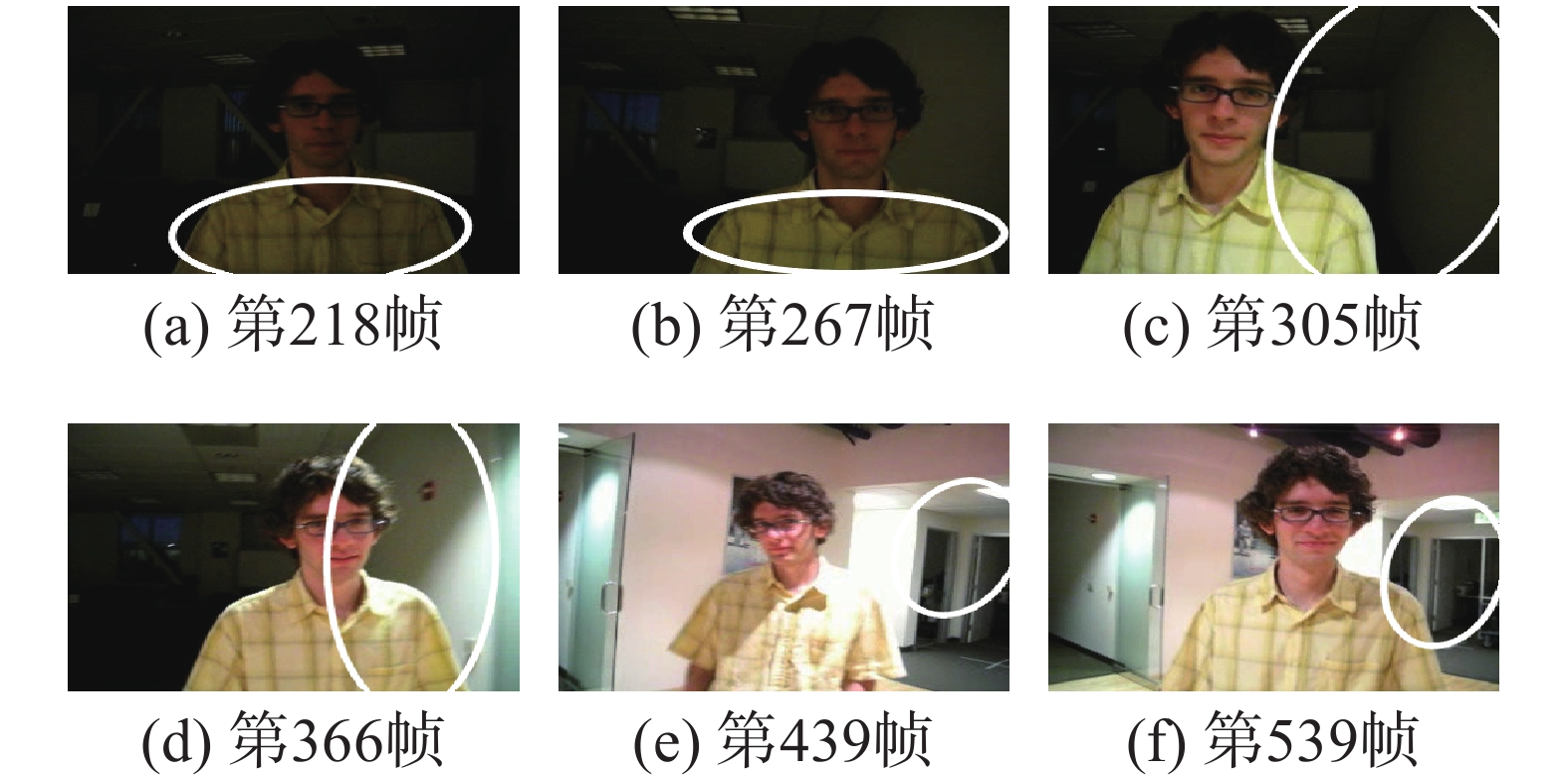Download: 图 14 多特征融合直方图模型跟踪效果 Fig. 14 Tracking result based on multiple features fusion histogram model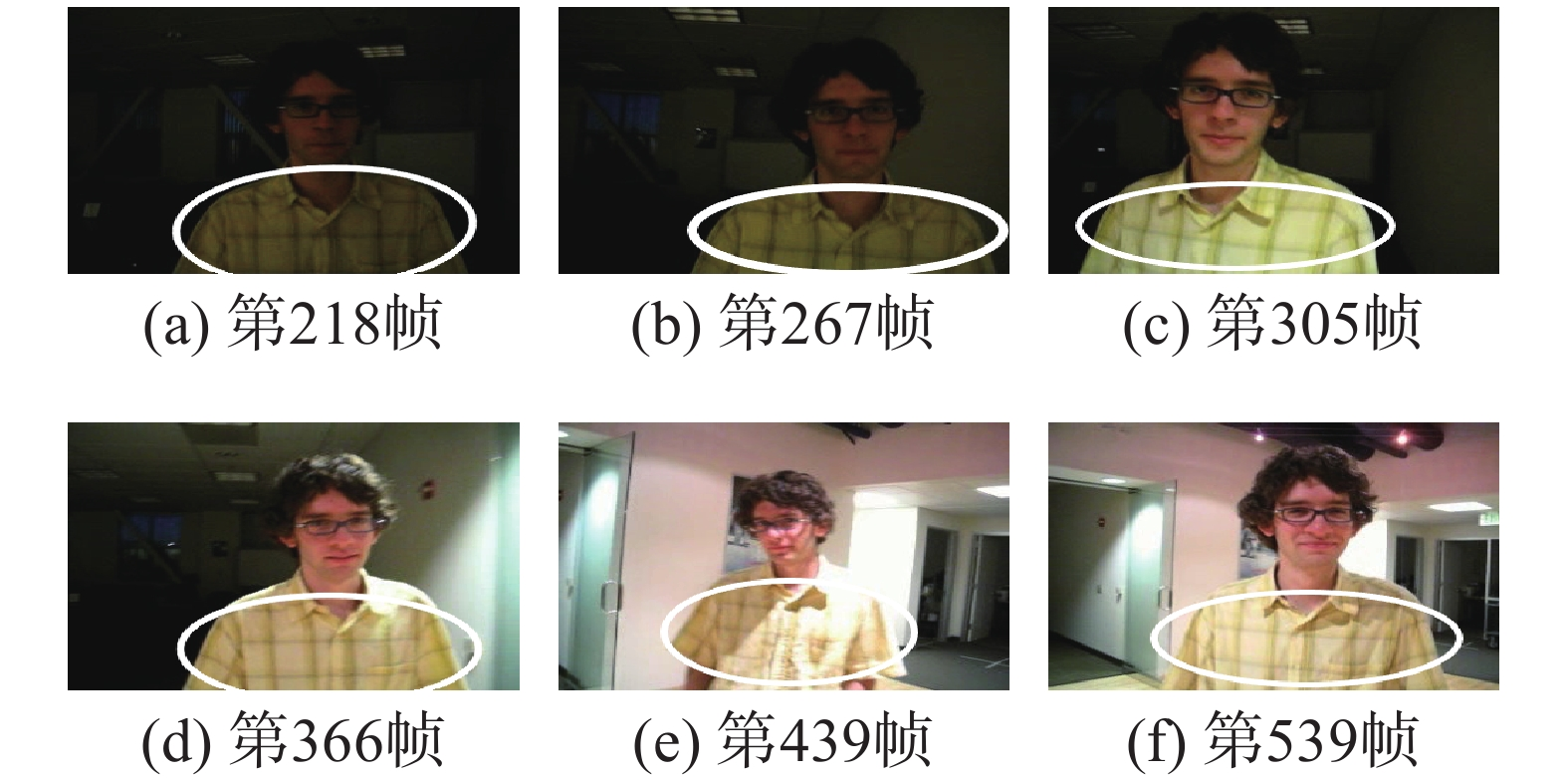Download: 图 15 模糊直方图模型跟踪效果 Fig. 15 Tracking result based on fuzzy histogram model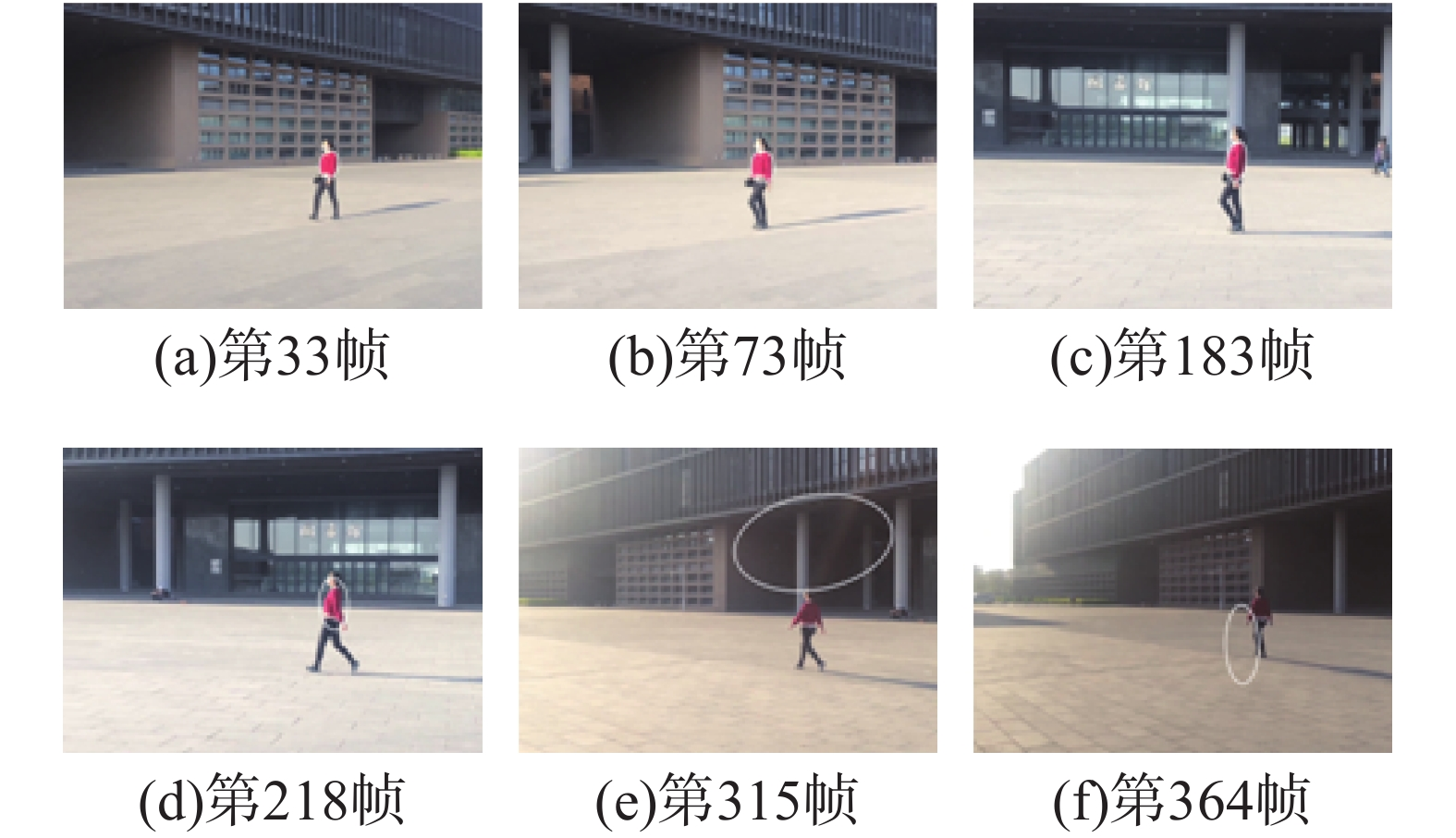Download: 图 16 传统直方图模型跟踪效果 Fig. 16 Tracking result based on the traditional histogram model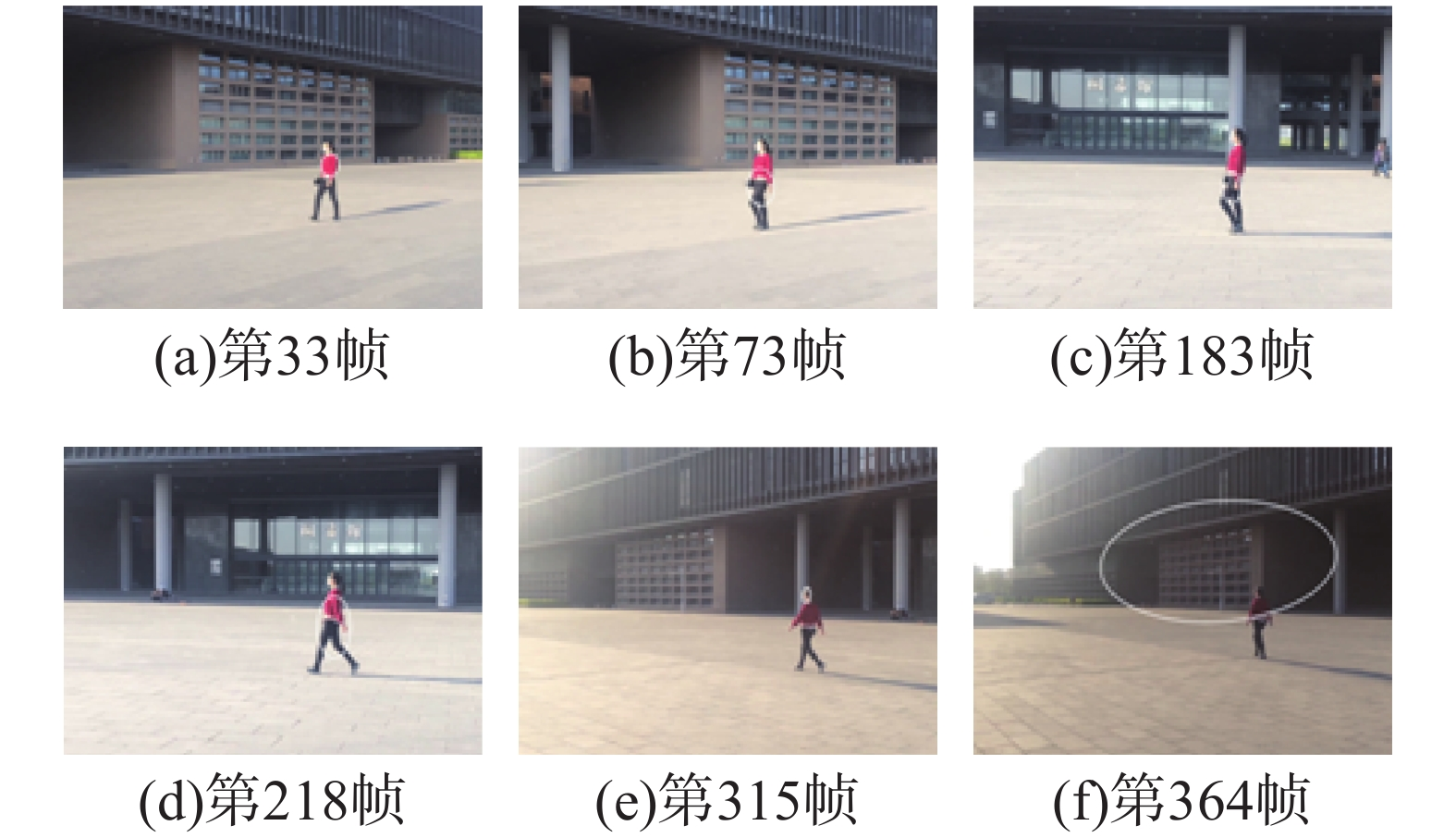Download: 图 17 显著性直方图模型跟踪效果 Fig. 17 Tracking result based on the saliency histogram model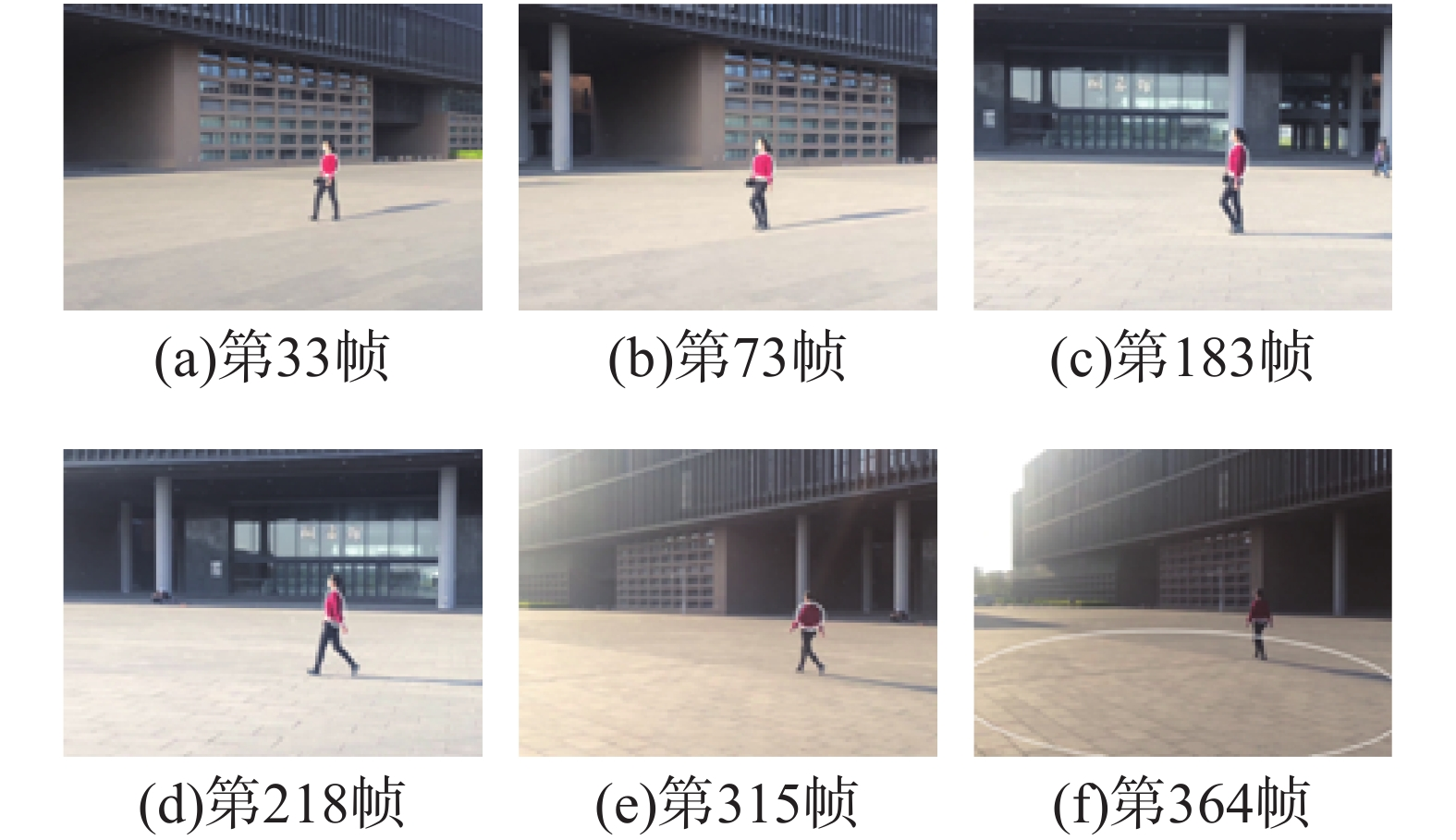Download: 图 18 多特征融合直方图模型跟踪效果 Fig. 18 Tracking result based on multiple features fusion histogram model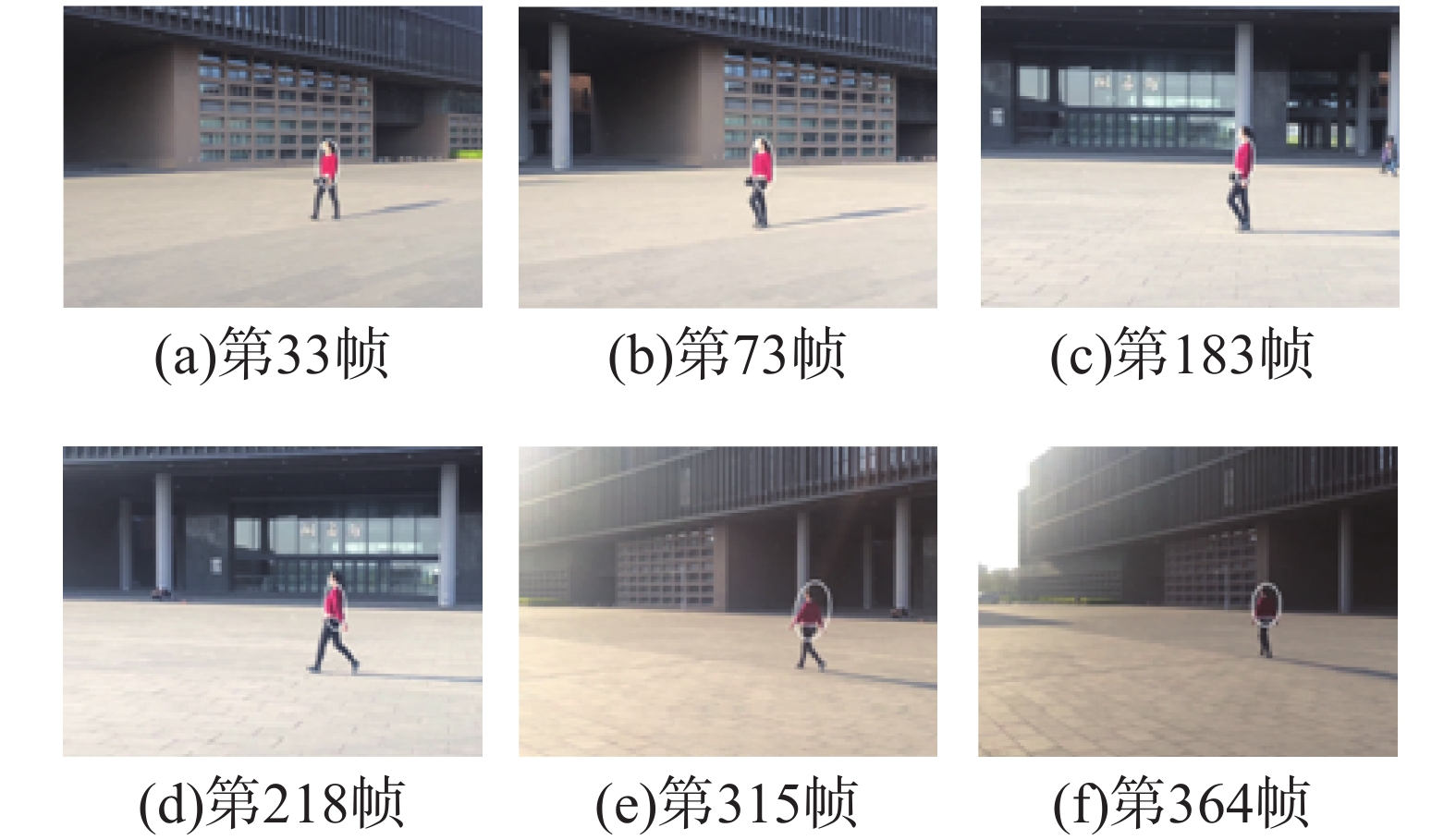Download: 图 19 模糊直方图模型跟踪效果 Fig. 19 Tracking result based on fuzzy histogram model

3.3 性能分析表 1 单帧跟踪对比时间 Tab.1 Tracking time of the single frame

4 结束语

  QU Shiru, LIU Ningning. Face detection and tracking method based on SMQT and CAMSHIFT[J]. Journal of computational information systems, 2015, 11(14): 5153-5162. (0)  黄晋英, 宋国浩, 兰艳亭, 等. 交比不变的Camshift跟踪方法[J]. 光学精密工程, 2016, 24(4): 945-953. HUANG Jinying, SONG Guohao, LAN Yanting, et al. Camshift tracking based on constant cross ratio[J]. Optics and precision engineering, 2016, 24(4): 945-953. (0)  刘威, 陈先桥, 初秀民. 基于置信规则推理方法的雷达目标跟踪[J]. 哈尔滨工程大学学报, 2016, 37(6): 826-831. LIU Wei, CHEN Xianqiao, CHU Xiumin. Radar target tracking via belief rule-based methodology[J]. Journal of Harbin Engineering University, 2016, 37(6): 826-831. (0)  王春平, 王暐, 刘江义, 等. 基于色度饱和度−角度梯度直方图特征的尺度自适应核相关滤波跟踪[J]. 光学精密工程, 2016, 24(9): 2293-2301. WANG Chunping, WANG Wei, LIU Jiangyi, et al. Scale adaptive kernelized correlation filter tracking based on HHS-OG feature[J]. Optics and precision engineering, 2016, 24(9): 2293-2301. (0)  朱齐丹, 韩瑜, 蔡成涛. 全景视觉非线性核相关滤波目标跟踪技术[J]. 哈尔滨工程大学学报, 2018, 39(7): 1220-1226. ZHU Qidan, HAN Yu, CAI Chengtao. Omni-directional visual object tracking using nonlinear kernelized correlation filters[J]. Journal of Harbin Engineering University, 2018, 39(7): 1220-1226. (0)  LIU Shenshen, ZHOU Pu, MA Chanyu. Athlete tracking model based on morphological noise removal camshift algorithm[J]. International journal of earth science and engineering, 2015, 8(5): 2246-2251. (0)  于蕾, 夏业儒, 杨良洁. 改进理解诊断跟踪系统的目标跟踪方法[J]. 应用科技, 2018, 45(4): 76-81. YU Lei, XIA Yeru, YANG Liangjie. A target tracking algorithm for the improved understanding, diagnosing and tracking system[J]. Applied science and technology, 2018, 45(4): 76-81. (0)  YIN Minghao, ZHANG Jin, SUN Hongguang, et al. Multi-cue-based CamShift guided particle filter tracking[J]. Expert systems with applications, 2011, 38(5): 6313-6318. DOI:10.1016/j.eswa.2010.11.111 (0)  HUANG Danchi, LI Lijuan. Face tracking algorithm based on improved Camshift and surf algorithm[J]. Journal of computational information systems, 2015, 11(3): 893-901. (0)  HSIA C H, LIOU Y J, CHIANG J S. Directional prediction camshift algorithm based on adaptive search pattern for moving object tracking[J]. Journal of real-time image processing, 2016, 12(1): 183-195. DOI:10.1007/s11554-013-0382-x (0)  修春波, 卢少磊, 任晓. 基于微分信息融合的Mean Shift改进跟踪算法[J]. 系统工程与电子技术, 2014, 36(5): 1004-1009. XIU Chunbo, LU Shaolei, REN Xiao. Improved mean shift tracking algorithm based on differential information[J]. Systems engineering and electronics, 2014, 36(5): 1004-1009. DOI:10.3969/j.issn.1001-506X.2014.05.31 (0)  修春波, 魏世安, 万蓉凤. 二维联合特征模型的自适应均值漂移目标跟踪[J]. 光电子·激光, 2015, 26(2): 342-351. XIU Chunbo, WEI Shian, WAN Rongfeng. CamShift target tracking based on two-dimensional joint characteristics[J]. Journal of optoelectronics·laser, 2015, 26(2): 342-351. (0)  张天翼, 杨忠, 韩家明, 等. 基于连续自适应均值漂移和立体视觉的无人机目标跟踪方法[J]. 应用科技, 2018, 45(2): 55-59. ZHANG Tianyi, YANG Zhong, HAN Jiaming, et al. Approach of vision navigation of UAV based on continuously adaptive mean-shift and stereo vision[J]. Applied science and technology, 2018, 45(2): 55-59. (0)  刘明华, 汪传生, 王宪伦. 基于多特征自适应融合的均值迁移目标跟踪算法[J]. 光电子·激光, 2015, 26(8): 1583-1592. LIU Minghua, WANG Chuansheng, WANG Xianlun. Mean-shift target tracking algorithm based on adaptive multi-features fusion[J]. Journal of optoelectronics·laser, 2015, 26(8): 1583-1592. (0)  夏瑜, 吴小俊, 李菊, 等. 基于多特征自适应融合的分类采样跟踪算法[J]. 光电子·激光, 2016, 27(3): 325-331. XIA Yu, WU Xiaojun, LI Ju, et al. Classified sampling tracking algorithm based on adaptive multiple features fusion[J]. Journal of optoelectronics·laser, 2016, 27(3): 325-331. (0)  杨超, 蔡晓东, 王丽娟, 等. 一种改进的CAMshift跟踪算法及人脸检测框架[J]. 计算机工程与科学, 2016, 38(9): 1863-1869. YANG Chao, CAI Xiaodong, WANG Lijuan, et al. An improved CAMShift tracking algorithm and a face detection framework[J]. Computer engineering and science, 2016, 38(9): 1863-1869. DOI:10.3969/j.issn.1007-130X.2016.09.019 (0)  修春波, 魏世安. 显著性直方图模型的Camshift跟踪方法[J]. 光学精密工程, 2015, 23(6): 1749-1757. XIU Chunbo, WEI Shian. Camshift tracking with saliency histogram[J]. Optics and precision engineering, 2015, 23(6): 1749-1757. (0)  林建华, 刘党辉, 邵显奎. 多特征融合的Camshift算法及其进一步改进[J]. 计算机应用, 2012, 32(10): 2814-2816, 2820. LIN Jianhua, LIU Danghui, SHAO Xiankui. Multi-feature fusion Camshift algorithm and its further improvement[J]. Journal of computer applications, 2012, 32(10): 2814-2816, 2820. (0)